Continuity Equation

 |   Tiled Menu   |   Tables of Content   |  Banner Video   |  Tohline Home Page   |

Various Forms

Lagrangian Representation

Among the principal governing equations we have included the

Standard Lagrangian Representation
of the Continuity Equation,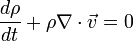$\frac{d\rho}{dt} + \rho \nabla \cdot \vec{v} = 0$

[BLRY07], p. 13, Eq. (1.53)

Note that this equation also may be written in the form,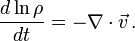$\frac{d \ln \rho}{dt} = - \nabla\cdot \vec{v} \, .$

Eulerian Representation

By replacing the so-called Lagrangian (or "material") time derivative,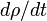$~d\rho/dt$, in the first expression by its Eulerian counterpart (see, for example, the wikipedia discussion titled, "Material_derivative," to understand how the Lagrangian and Eulerian descriptions of fluid motion differ from one another conceptually as well as how to mathematically transform from one description to the other), we directly obtain what is commonly referred to as the

Eulerian Representation
or
Conservative Form
of the Continuity Equation,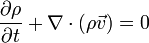$~\frac{\partial\rho}{\partial t} + \nabla \cdot (\rho \vec{v}) = 0$

[BLRY07], p. 7, Eq. (1.24)

Time-independent Behavior

Lagrangian Frame of Reference

If you are riding along with a fluid element — viewing the system from a Lagrangian frame of reference — the mass density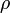$~\rho$ of your fluid element will, by definition, remain unchanged over time if,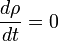$\frac{d\rho}{dt} = 0$ .

From the above "Standard Lagrangian Representation" of the continuity equation, this condition also implies that,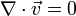$\nabla\cdot \vec{v} = 0$ .

Looking at it a different way, if while riding along with a fluid element you move through a region of space where$\nabla\cdot \vec{v} = 0$, your mass density will remain unchanged as you move through this region.

Eulerian Frame of Reference (steady-state mass distribution)

On the other hand, if you are standing at a fixed location in your coordinate frame watching the fluid flow past you — viewing the system from an Eulerian frame of reference — the mass density of the fluid at your location in space will, by definition, always be the same if,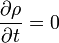$\frac{\partial\rho}{\partial t} = 0$ .

From the above "Eulerian Representation" of the continuity equation, this condition also implies that a steady-state mass distribution will be governed by the relation,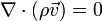$\nabla\cdot (\rho \vec{v}) = 0$ .

 © 2014 - 2019 by Joel E. Tohline |   H_Book Home   |   YouTube   | Context: | PGE | SR | Appendices: | Equations | Variables | References | Binary Polytropes | Ramblings | Images | Images (2016 Layout) | ADS |